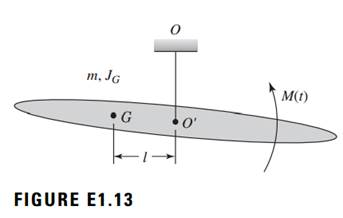### Create an Account

Already have account?

### Forgot Your Password ?

Home / Questions / Consider the rigid body shown in Figure E113 This body has a mass m and rotary inertia JG ...

# Consider the rigid body shown in Figure E113 This body has a mass m and rotary inertia JG about the center of mass G It is suspended from a point O on the ceiling by using an elastic

Consider the rigid body shown in Figure E1.13. This body has a mass m and rotary inertia JG about the center of mass G. It is suspended from a point O on the ceiling by using an elastic suspension. The point of attachment O is at a distance l from the center of mass G of this body. M(t) is an external moment applied to the system along an axis normal to the plane of the body. Use the generalized coordinates x, which describes the up and down motions of point O from point O, and u, which describes the angular oscillations about an axis normal to the plane of the rigid body. For the system shown in Figure E1.13, use the principle of angular-momentum balance given and obtain an equation of motion for the system. Assume that gravity loading is present.Jun 25 2020 View more View Less

#### Answer (Solved)Subscribe To Get Solution